## 2. An alternating current is represented by the equation I=20sin 100mt. Compute its frequency and the maximum r.m.s values of current.<

Question

2. An alternating current is represented by the equation I=20sin 100mt.
Compute its frequency and the maximum r.m.s values of current.
Ans: 50Hz,20A,14.14A

in progress 0
2 weeks 2021-09-03T17:31:28+00:00 1 Answers 0 views 0

1. Explanation:

The general equation of an AC current is given by :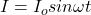Where

I₀ is the peak value of current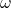is angular frequency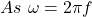So,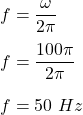We know that,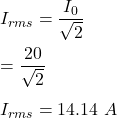So, the frequency is 50 Hz and the maximum rms value of current is 14.14 A.